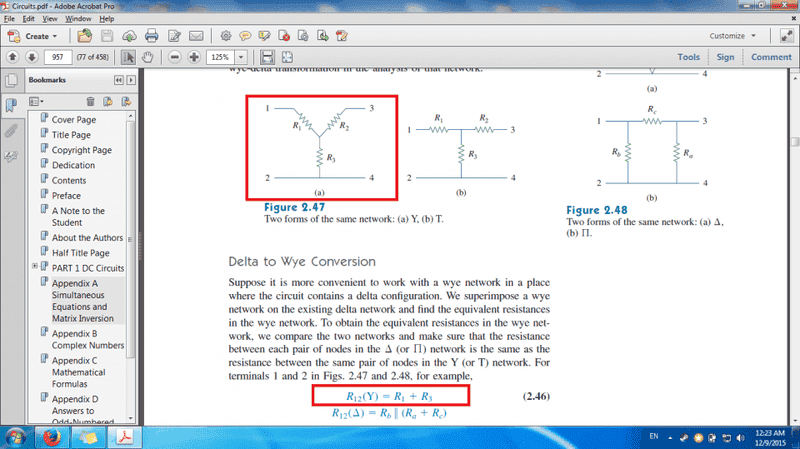# Proof of Wye-Delta Transform Doesn't Make Sense

In the picture taken from my book, in the bottom red box, it states that the equivalent resistance seen between terminals 1 and 2 is R1 + R3, implying R1 and R3 are in series.

But clearly, there is a third resistor R3 at the same node where R1 and R2 meet. Then that means R1 and R3 cannot be in series. Then why does the book state the equivalent resistance is R1+R3?

#### Attachments

•Untitled.png
98.1 KB · Views: 569

cnh1995
Homework Helper
Gold Member
Then that means R1 and R3 cannot be in series. Then why does the book state the equivalent resistance is R1+R3?
You need to understand the meaning of equivalent resistance. Here, R12 is the equivalent resistance between 1 and 2. This means if there were a source connected between 1 and 2, it would "see" the resistance of the network as R12. Hence, R1 and R3 are in series and R2 is redundant.

I don't think that's correct, that they're in series. If they were in series, Both R1 and R3 would have the same current passing through them. But if you pass a current downwards from R1, and downwards from R2, R3 gets the addition of both currents, not just from R1. No matter how I arrange the currents, I can't see any way for resistors R1 and R3 to have the same current, and hence be in series, unless R2 is disconnected.

SammyS
Staff Emeritus
Homework Helper
Gold Member
I don't think that's correct, that they're in series. If they were in series, Both R1 and R3 would have the same current passing through them. But if you pass a current downwards from R1, and downwards from R2, R3 gets the addition of both currents, not just from R1. No matter how I arrange the currents, I can't see any way for resistors R1 and R3 to have the same current, and hence be in series, unless R2 is disconnected.When they refer to the equivalent resistance between terminals #1 and #2, that implies that the are no connections to the other two terminals. Therefore, for that euivalent resistance, resistor, R2 is not involved at all. The only path for current to flow would be through resistors, R1 and R3.

sophiecentaur
Gold Member
2020 Award
@yosimba200 If you have doubts about this then you could always put in some values, go through the calculations and see what you get. If the answers agree then, at least, you can believe the system works ('cos it does). You could then be 'emotionally OK' with the theory; intuition can often get in the way of understanding.
What I am suggesting involves putting a voltage source on the left and a resistive load on the right and working out the current into the load (in both cases, using the formulae to get the WYE values from the DELTA). If you don't like the sums then use a simulation to get your answer (or some resistors and a soldering iron)

Wait a minute....

The resistance measured in by the probes of an ohm-meter calculate only the resistors that are connected between the probes, right?

cnh1995
Homework Helper
Gold Member
Wait a minute....

The resistance measured in by the probes of an ohm-meter calculate only the resistors that are connected between the probes, right?
Yes. It measures the equivalent resistance between those two points.

sophiecentaur